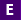#Not a Florida public school educator?

Access this resource
on CPALMS.com

### General Information

Subject(s): Mathematics, Science
Intended Audience: EducatorsInstructional Time: 40 Minute(s)
Keywords: proportional relationships, unit rate, slope, slope intercept, graph proportional relationship, interpreting unit rate, density, density of materials, mass, volume, computer science, computer, coding, scratch, graphing, constant of proportionality, proportionality constant, slope of the line, compare slopes
Instructional Component Type(s):

## Aligned Standards

This vetted resource aligns to concepts or skills in these benchmarks.

## 2 Lesson Plans

Slope Intercept - Lesson #2

This is lesson 2 of 3 in the Slope Intercept unit. This lesson introduces graphing non-proportional linear relationships. In this lesson students will perform an activity to collect data to derive y = mx + b and will use a Scratch program to plot the graph of the data, as well as check for proportional and/or linear relationships.

Slope Intercept - Lesson #3

This is lesson 3 of 3 in the Slope Intercept unit. This lesson introduces similar triangles to explain why slope is the same between any two points on a non-vertical line. In this lesson students perform an activity to determine that slope is constant throughout a line and students will discover the slope for vertical and horizontal lines.

## Related Resources

Other vetted resources related to this resource.

## Lesson Plans

Slope Intercept - Lesson #3:

This is lesson 3 of 3 in the Slope Intercept unit. This lesson introduces similar triangles to explain why slope is the same between any two points on a non-vertical line. In this lesson students perform an activity to determine that slope is constant throughout a line and students will discover the slope for vertical and horizontal lines.

Type: Lesson Plan

Slope Intercept - Lesson #2:

This is lesson 2 of 3 in the Slope Intercept unit. This lesson introduces graphing non-proportional linear relationships. In this lesson students will perform an activity to collect data to derive y = mx + b and will use a Scratch program to plot the graph of the data, as well as check for proportional and/or linear relationships.

Type: Lesson Plan